# Lim-Eom 3-way Power Splitters

Click here to go to our main page on couplers and splitters

We now have two examples of using Lim and Eom's unique splitter to combiner power amplifiers:

Click here to learn how to use the Lim Eom splitter as a three-way combiner

Click here to learn how to use the Lim Eom splitter as a two-way combiner

This is a truly new power splitter (a rare event in microwaves!), first detailed by Jong-Sik Lim and Soon-Young EOM in a 1996 IEEE MTT-S paper titled A New 3-way Power Divider with Various Output Ratios. As a three-way splitter, it fits only niche applications, because of the phase relationships of the three outputs, as well as the asymmetric layout, but still, it's a cool splitter that you should consider. One application that comes to mind in the LO splitter for the classic three-channel monopulse receiver. By the way, Lim and Eom were at the Seoul National University's Applied Electromagnetics Laboratory when they wrote the origianl paper. The AEL posts many of their publications on their web site, definitely worth checking out! We have a page on SNU go there if you want to check out the link!

We made a model of Lim and Eom's six-port neteowrk using Eagleware Genisys. We also entered the equations into Excel, just to play with the split ratios to see the effect on line impedances.

The splitter resembles a double-box hybrid. Below is the schematic from our Genisys project. Port 1 (top left port) is the common port, while ports 2, 4 and 6 are the split ports. Note that you have to terminate ports 3 and 5 to operate it. The line impedances shown will provide an equal three-way split in a fifty ohm system.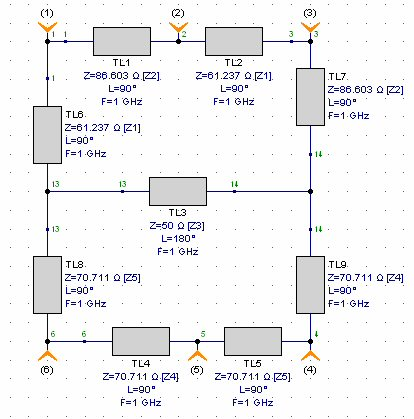Lim and EOM's paper gives equations for line impedances that you can use to obtain different power split ratios. The integers m, n and k are what sets up the power division. For an equal split, m=n=k=1. The impedances given in the above schematic are for equal split. Here is how we entered them in Genisys:

m=?1
n=?1
k=?1
delta1=m+n+k
delta2=n+k
Z0=50
Z1=(delta1/delta2)^.5*Z0
Z2=(delta1/m)^.5*Z0
Z3=Z0
Z4=(delta2/N)^.5*Z0
Z5=(delta2/K)^.5*Z0

These are the variables that Lim and EOM defined in their paper. Note that m, n, k don't have to be integers (and in Genisys, the question mark is what allows you to tune them while viewing the response). It is easy to convert the equations so that you input the ratios in dB (that's the first thing we did, in an Excel file we'll give you if you ask nicely!)

Note that the impedance Z3 is has no effect on the power split. We left it at 50 ohms like they did in their paper. The plot below shows the frequency response for the equal split case (-4.77 dB each arm). Notice that the bandwidths of the three split arms are all different. The bandwidth isn't all that good relative to a Wilkinson splitter.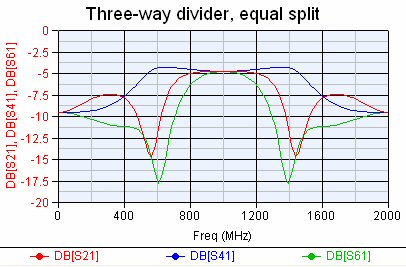Below is the isolation of ports 3, and 5, along with the input match S11. From this point of view you could use the splitter over a 20% bandwidth and get 20 dB isolation.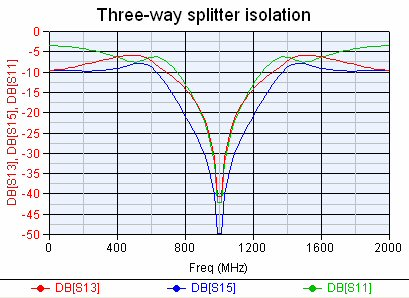Let's look at the phases of each path. The longest path is S14 (one wavelength), followed by S16 (half-wavelength), then S12 (quarter-wavelength). We see that at center frequency that S14 and S16 are +/- 90 degrees out of phase with S12, and the phase relationship is not very constant over frequency. This might be a limitation in certain applications, for equal phases you should consider a three-way Wilkinson. You might think of the Lim-Eom splitter as a combination of a 90 degree hybrid and a 180 degree hybrid.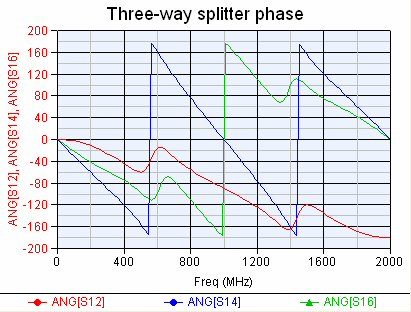If you wanted to achieve equal phase, equal split, here's a layout for you. The input port is in the center of the north side, and the west, south and east sides have transmission lines that give nearly equal phase outputs. Note the "fan stub" terminations, whoever did this cool design deserves a fat raise!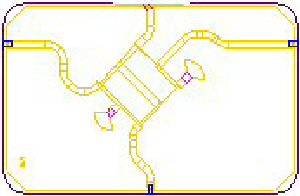Now let's try out an unequal split, in this case 1:5:5. You might do this if you wanted most of your power to feed two devices, and the third port going to a detector to monitor power. We followed the equations and they do what Lim and EOM claimed! The two facored ports are 7 dB above the starved port.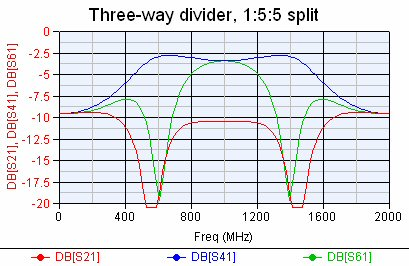More to come! We still need to look at line impedances versus coupling ratios.

Author : Unknown Editor